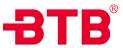• 系列号
A=角座阀
• 功能
7=常闭
8=常开
9=双作用
10=自由态
• 阀体材质
1=316L
2=316
3=304
• 执行器材质
3=工程塑料
4=铝合金
5=304不锈钢
• 主要密封材质
9=PTFE(-20℃ ~ +180℃)
1=PTFE复合(-20℃ ~ +220℃)
• 执行器尺寸
04=40mm
05=50mm
06=63mm
08=80mm
10=100mm
12=125mm
15=150mm
• 接口
G=英制直管螺纹BSP DIN ISO228-1(国内G)
T=英制锥管螺纹BSPT DIN2999-1(国内Rc)
N=美制锥管螺纹NPTASMEB1.20.1
K=快装式ISO2852
K2=快装式定制尺寸
F=法兰式HG5010-58
F2=法兰式定制尺寸
H1=焊接式DIN11850-
H2=焊接式DIN11850-2
H3=焊接式DIN11850-3
H4=焊接式ISO 4200
H5=焊接式DIN3239
H6=焊接式ASME BPE
H7=焊接式ISO037
H8=齐面倒角
• 规格
-15=1/2"（DN15）
-20=3/4" (DN20)
-25=1"(DN25)
-32=1 1/4"(DN32)
-40=1 1/2"(DN40)
-50=2"(DN50)
-65=2 1/2"(DN65)
-80=3"(DN80)
-100=4"(DN100)
• 附件
-F3=两位三通电磁阀
-F4=两位五通电磁阀
-F5=双位反馈器
-F6=线式信号反馈器：a-高频 b-低频
• 流向
-A=A流向（承压高）
-B=B流向（无水锤）